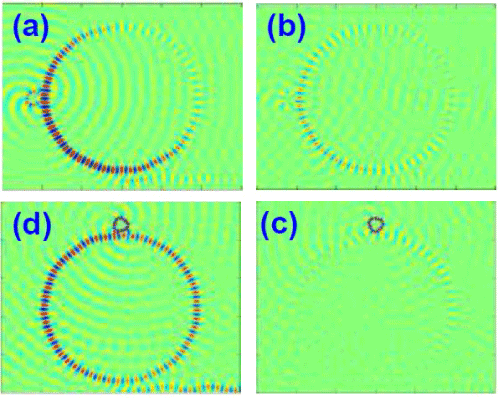Figure 4: Light intensity in time-domain in microring sensor (R=10 μm, dR=1μm, n=1.46). First row: light couple non-resonantly to SO (r=1micron, n=2.0) having no eigenmodes close to the light frequencies (a) at time t = 0.8 ps, and (b) at time t=2.4 ps. Second row: light couple resonantly to SO (r=1micron,n=3.0) having eigenmodes close to the light frequencies (c) at time t=0.8 ps,and (d) at time t=2.4 ps.
Goto home»